# Texas Go Math Grade 2 Lesson 15.3 Answer Key Compose Three-Dimensional Solids

Refer to our Texas Go Math Grade 2 Answer Key Pdf to score good marks in the exams. Test yourself by practicing the problems from Texas Go Math Grade 2 Lesson 15.3 Answer Key Compose Three-Dimensional Solids.

## Texas Go Math Grade 2 Lesson 15.3 Answer Key Compose Three-Dimensional Solids

Explore

Circle the solids with curved surfaces. Draw an X on the solids with flat surfaces.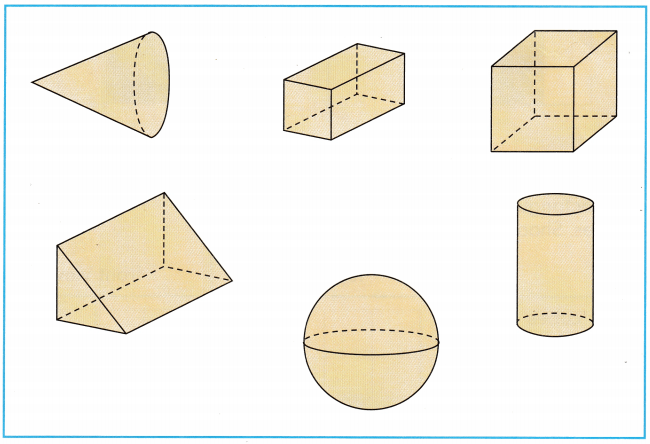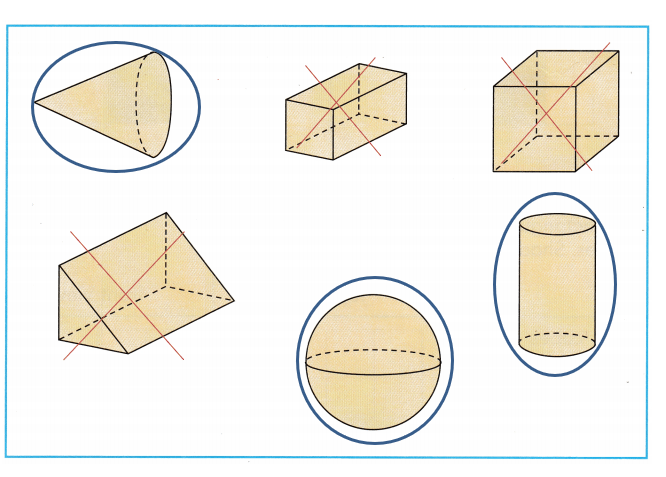HOME CONNECTION • Your child sorted the solids on this page using the attributes of the solids.

Sphere has no faces, edges, and vertex

Cone has 2 faces, 1 edge, and 1 vertex

The cylinder has 3 faces, 3 edges, and 0 vertex

The triangular prism has 5 faces, 9 edges, and 6 vertices

Cube has 6 faces, 12 edges, and 8 vertices

The rectangular prism has 6 faces, 12 edges, and 8 vertices

Math Talk
Mathematical Processes

Name the shapes you drew an X on. Describe how they are different.
The rectangular prism has 6 faces, 12 edges, and 8 vertices

The triangular prism has 5 faces, 9 edges, and 6 vertices

Cube has 6 faces, 12 edges, and 8 vertices

Model and Draw

Build this rectangular prism using 12 unit cubes.The shading shows the top and front views.
top viewfront view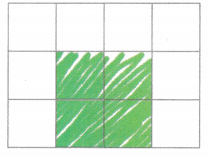Share and Show

Build a rectangular prism with the given number of unit cubes. Shade to show the top and front views.

Question 1.
9 unit cubes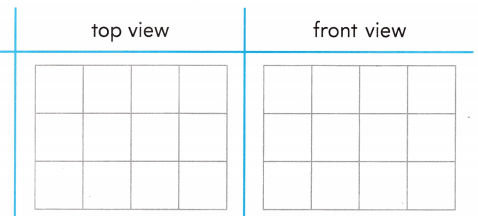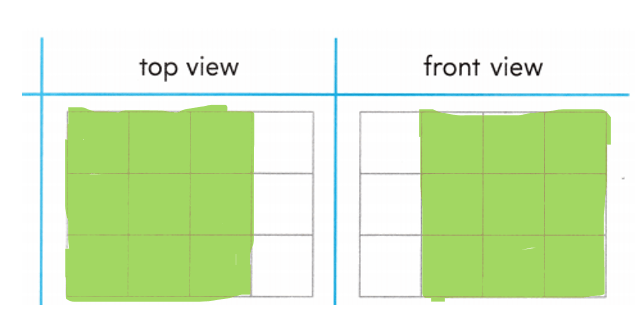Question 2.
16 unit cubes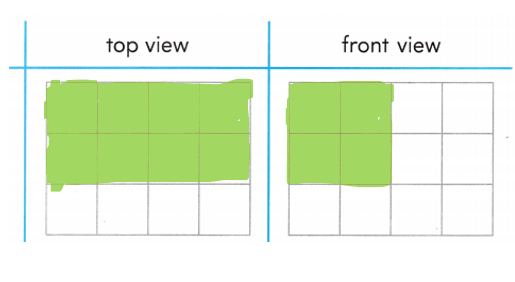Problem Solving

Build a rectangular prism with the given number of 7 unit cubes. Shade to show the top and front views.

Question 3.
24 unit cubes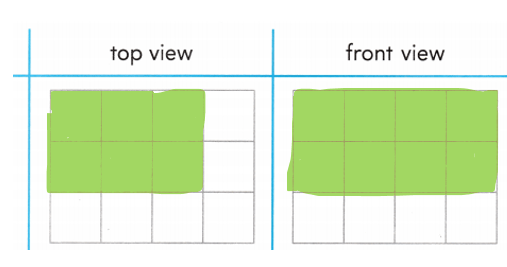Question 4.
H.O.T. Multi-Step The top, side, and front views of a rectangular prism are shown. Build the prism. How many unit cubes are used to build the solid?___________ unit cubes
18 cube units

Explanation:
Volume = length × width × height

= 3 × 2 × 3

= 18 cube units

Question 5.
H.O.T. Jen uses 18 cubes to build a rectangular prism. The top and front views are shown. Shade to show the side view.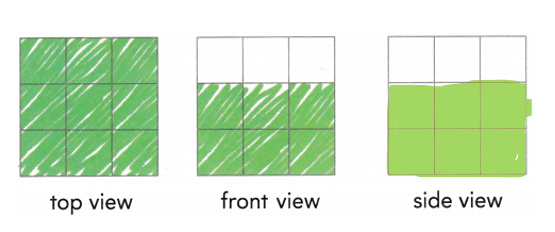Explanation:

Volume = length × width × height

= 3 × 2 × 3

= 18 cube units

Question 6.
Apply Theo builds the first layer of a prism using 4 cubes. He adds 3 more layers of 4 cubes each. How many cubes are used for the prism?
(A) 7
(B) 16
(C) 12
16

Explanation:
Theo builds the first layer of prism = 4 cubes
then he adds 3 more layers = 3 × 4 = 12
Then he adds 4 cubes each = 12 + 4 = 16

Question 7.
Analyze Dan built this rectangular prism. How many unit cubes did he use?(A) 18
(B) 10
(C) 24
24 unit cubes

Explanation:
Volume = length × width × height

=   3 × 2 × 4

=  24

Question 8.
Multi-Step Ella builds the first layer of a rectangular prism using 6 cubes. James adds 2 more layers of 6 cubes each. How many cubes are used for the prism?
(A) 8
(B) 18
(C) 12
18

Explanation:
Ella builds first layer = 6 cubes

James adds 2 more layers = 6 × 2 = 12

2 layers of 6 cubes each = 12 + 6 = 18

Question 9.
Texas Test Prep Tyler built a rectangular prism using unit cubes. How many unit cubes did Tyler use?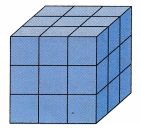(A) 27
(B) 9
(C) 18
27 cube units

Explanation:
Number of cubes in each layer = 9

Number of layers = 3

Number of cube units = 9 × 3

= 27 cube units

TAKE HOME ACTIVITY • Ask your child to show how he or she solved on exercise in the lesson.

### Texas Go Math Grade 2 Lesson 15.3 Homework and Practice Answer Key

Shade to show the top and front views of each rectangular prism.

Question 1.Question 2.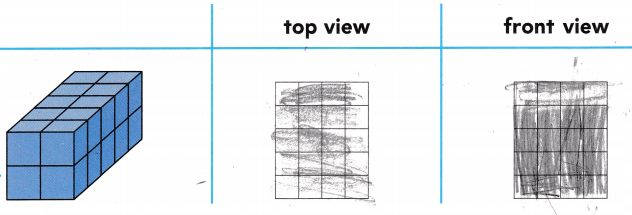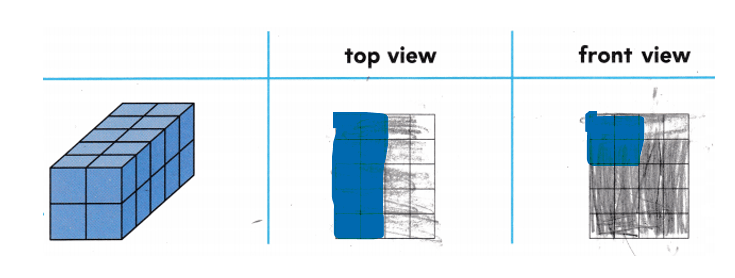Problem Solving

Question 3.
The top, side, and front views of a rectangular prism are shown. How many unit cubes are used to build the solid?__________ cubes
18 cube units

Explanation:

Volume = length × width × height

= 3 × 2 × 3

= 18 cube units

Lesson Check

Question 4.
Ken built a rectangular prism using unit cubes. How many unit cubes did Ken use?(A) 24
(B) 8
(C) 12
24 cube units

Explanation:
Volume = length × width × height

= 4 × 2 × 3

= 24

Question 5.
Multi-Step Abby builds the first layer of a rectangular prism using 6 cubes. Liam adds 2 more layers. How many cubes are used for the prism?
(A) 7
(B) 18
(C) 10
18

Explanation:
Number of cubes Abby builds first layer=6
Liam add 2 more layers= base cubes + 2nd layer cubes + 3rd layer cubes

Liam add 2 more layers= 6 + 6 + 6
= 18

Question 6.
Colby builds the first layer of a rectangular prism using 8 cubes. He adds another layer of cubes. How many cubes are used for the prism?
(A) 9
(B) 8
(C) 16
16

Explanation:
Number of cubes Colby builds the first layer of a rectangular prism = 8

He adds another layer of cubes = base layer + another layer

cubes used = 6 +6

cubes used = 12

Question 7.
Erica builds the first layer of a rectangular prism using 2 cubes. She adds 3 more layers. How many cubes are used for the prism?
(A) 4
(B) 8
(C) 6